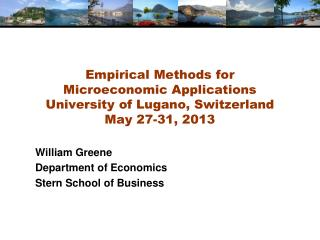DownloadDownload PresentationWilliam Greene Department of Economics Stern School of Business

# William Greene Department of Economics Stern School of Business

Télécharger la présentation## William Greene Department of Economics Stern School of Business

- - - - - - - - - - - - - - - - - - - - - - - - - - - E N D - - - - - - - - - - - - - - - - - - - - - - - - - - -
##### Presentation Transcript

1. Empirical Methods for Microeconomic ApplicationsUniversity of Lugano, SwitzerlandMay 27-31, 2013 William Greene Department of Economics Stern School of Business

2. 2A. Models for Count Data, Inflation Models

3. Agenda for 2A • Count Data Models • Poisson Regression • Overdispersion and NB Model • Zero Inflation • Hurdle Models • Panel Data

4. Doctor Visits

5. Basic Model for Counts of Events E.g., Visits to site, number of purchases, number of doctor visits Regression approach Quantitative outcome measured Discrete variable, model probabilities Nonnegative random variable Poisson probabilities – “loglinear model”

6. Poisson Model for Doctor Visits

7. Alternative Covariance Matrices

8. Partial Effects

9. Poisson Model Specification Issues Equi-Dispersion: Var[yi|xi] = E[yi|xi]. Overdispersion: If i = exp[’xi + εi], E[yi|xi] = γexp[’xi] Var[yi] > E[yi] (overdispersed) εi ~ log-Gamma  Negative binomial model εi ~ Normal[0,2]  Normal-mixture model εi is viewed as unobserved heterogeneity (“frailty”). Normal model may be more natural. Estimation is a bit more complicated.

10. Overdispersion • In the Poisson model, Var[y|x]=E[y|x] • Equidispersion is a strong assumption • Negbin II: Var[y|x]=E[y|x] + 2E[y|x]2 • How does overdispersion arise: • NonPoissonness • Omitted Heterogeneity

11. Negative Binomial Regression

12. NegBin Model for Doctor Visits

13. Negative Binomial Specification Prob(Yi=j|xi) has greater mass to the right and left of the mean Conditional mean function is the same as the Poisson: E[yi|xi] = λi=Exp(’xi), so marginal effects have the same form. Variance is Var[yi|xi] = λi(1 + α λi), α is the overdispersion parameter; α = 0 reverts to the Poisson. Poisson is consistent when NegBin is appropriate. Therefore, this is a case for the ROBUST covariance matrix estimator. (Neglected heterogeneity that is uncorrelated with xi.)

14. Testing for Overdispersion Regression based test: Regress (y-mean)2 on mean: Slope should = 1.

15. Wald Test for Overdispersion

16. Partial Effects Should Be the Same

17. Model Formulations for Negative Binomial E[yi |xi ]=λi

18. NegBin-1 Model

19. NegBin-P Model NB-2 NB-1 Poisson

20. Zero Inflation?

21. Zero Inflation – ZIP Models Two regimes: (Recreation site visits) Zero (with probability 1). (Never visit site) Poisson with Pr(0) = exp[- ’xi]. (Number of visits, including zero visits this season.) Unconditional: Pr = P(regime 0) + P(regime 1)*Pr[0|regime 1] Pr[j | j >0] = P(regime 1)*Pr[j|regime 1] This is a “latent class model”

22. Two Forms of Zero Inflation Models

23. An Unidentified ZINB Model

24. Notes on Zero Inflation Models • Poisson is not nested in ZIP. tau = 0 in ZIP(tau) or γ = 0 in ZIP does not produce Poisson; it produces ZIP with P(regime 0) = ½. • Standard tests are not appropriate • Use Vuong statistic. ZIP model almost always wins. • Zero Inflation models extend to NB models – ZINB(tau) and ZINB are standard models • Creates two sources of overdispersion • Generally difficult to estimate • Tau form is not a good model – not generally used

25. Partial Effects for Different Models

26. The Vuong Statistic for Nonnested Models

27. A Hurdle Model Two part model: Model 1: Probability model for more than zero occurrences Model 2: Model for number of occurrences given that the number is greater than zero. Applications common in health economics Usage of health care facilities Use of drugs, alcohol, etc.

28. Hurdle Model

29. Hurdle Model for Doctor Visits

30. Partial Effects

31. Winkelmann finds that there is no correlation between the decisions… A significant correlation is expected … [T]he correlation comes from the way the relation between the decisions is modeled.

32. Probit Participation Equation Poisson-Normal Intensity Equation

33. Bivariate-Normal Heterogeneity in Participation and Intensity Equations Gaussian Copula for Participation and Intensity Equations

34. Correlation between Heterogeneity Terms Correlation between Counte

35. Panel Data Models Heterogeneity; λit = exp(β’xit + ci) • Fixed Effects • Poisson: Standard, no incidental parameters issue • NB • Hausman, Hall, Griliches (1984) put FE in variance, not the mean • Use “brute force” to get a conventional FE model • Random Effects • Poisson • Log-gamma heterogeneity becomes an NB model • Contemporary treatments are using normal heterogeneity with simulation or quadrature based estimators • NB with random effects is equivalent to two “effects” one time varying one time invariant. The model is probably overspecified Random Parameters: Mixed models, latent class models, hiererchical – all extended to Poisson and NB

36. Random Effects